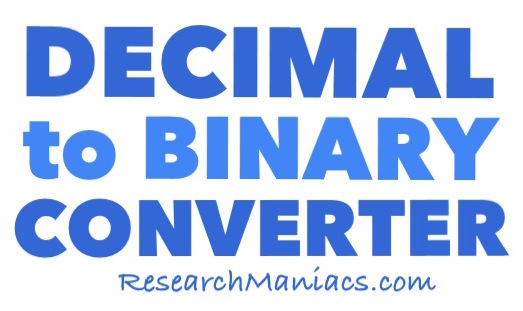How to Convert 8 Decimal to Binary

 Here are step-by-step instructions on how to convert the decimal number 8 to a binary number. Step A) Determine if 8 is an even or odd number. If it is even, you assign a 0 and if it is odd you assign a 1. Step B) Deduct the 1 or 0 in step A from 8 and then divide it by 2. Then, you see if that number is even or odd and then assign a 0 or 1 like step A. Step C) Repeat step B with the new number until the number you get is 1. Step D) Put all the 0s and 1s you assigned in reverse order to get the answer. To illustrate better, we detailed below how we converted 8 decimal to binary using the steps above. 8 → 0(8 - 0)/2 = 4 → 0(4 - 0)/2 = 2 → 0(2 - 0)/2 = 1 → 1 Then you put the binary number above in reverse order and you get the answer: 1000Convert Decimal to Binary Enter another decimal below to convert it to binary.

 How to Convert 9 Decimal to Binary On this page we showed you how to convert 8 decimal to binary. Use this knowledge to see if you can convert the next decimal number on our list to binary!